Like   Tweet   +1
 /* styles */ Issue 54, April 2018

## Wha gwaan?

 /* styles */ Noticing all the links in recent Whale's Tales? Now in light blue and easier to find - "Keep sailing Bob" will soon mean something to you. It's worth hovering over the pictures too. You will have received notification of our Presentation Night and Rescue Boat Training events. These are still ahead of us and do require responses so please book in early as it really helps those organising it for you. Thanks. Our harbour put on its best for the National E fleet holding their NSW champs on the 24th of March. And while a couple of pesky Victorians came to do battle, the trophy remains in the hands of locals. See full report below. Warning: QI section may contain traces of nuts but has inspired me to coin the term Navixanthoprasinophobia - let me know what you think this is. Boyd Newton, back half of 'Da Easy Crew, Editor.
 table div table+table+table+table+table div table{width:100%;padding:0}table div table+table+table+table+table div table img{width:96.23%;padding:0;float:none}table div table+table+table+table+table div table td{width:100%;padding:0 1.88% 18px}/* styles */## Important Info

Please look into the following as others may be relying on you to do so:

▪ Rescue Boat Training
▪ BSC Apparel
▪ Presentation Night
 ▪ Rescue Boat Training
 ▪ BSC Apparel
 ▪ Presentation Night

## Dubious Info

Be cautious out there, 3.14% of Sailors are Pi-rates.

## New Members

We are lucky to have three additions to the BSC family this month.

▪ Kristoff - ice harvester, always has best friend by his side.
▪ Sven - loyal companion of Kristoff.
▪ Duke of Weselton - lacks in stature but makes up for it in showboating.
 ▪ Kristoff - ice harvester, always has best friend by his side.
 ▪ Sven - loyal companion of Kristoff.
 ▪ Duke of Weselton - lacks in stature but makes up for it in showboating.

You'll find these cool characters upstairs at the clubhouse.

 table div table+table+table+table+table+table+table+table+table div table{width:100%;padding:0}table div table+table+table+table+table+table+table+table+table div table img{width:96.23%;padding:0;float:none}table div table+table+table+table+table+table+table+table+table div table td{width:100%;padding:0 1.88% 18px}/* styles */## A joint effort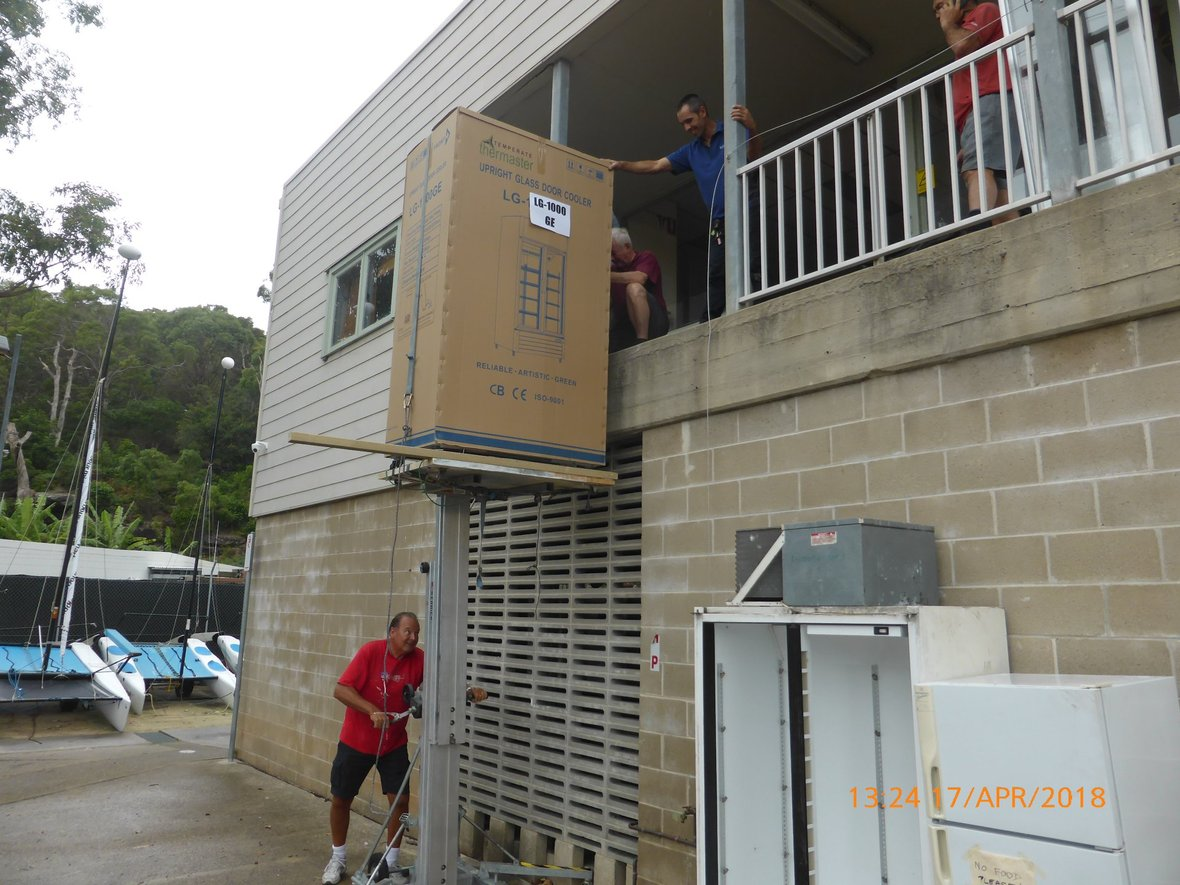Kristoff, Sven & the Duke of Weselton move in.

Thanks to John Stracey, Andrew and Ian Sim, Graham Hanna, Roly Webb and Stephen Johnco for installing two new fridges and a freezer in the clubhouse and removing the old ones.

Judging by the photo this was no easy feat. A job very well done lads.

## Know someone we should recognise?

Email Boyd details of someone quietly working away behind the scenes.

 table div table+table+table+table+table+table+table+table+table+table+table+table+table+table div table{width:100%;padding:0}table div table+table+table+table+table+table+table+table+table+table+table+table+table+table div table img{width:96.23%;padding:0;float:none}table div table+table+table+table+table+table+table+table+table+table+table+table+table+table div table td{width:100%;padding:0 1.88% 18px}/* styles */## BSC is holding training on Saturday 30th June 2018

This full day event will cover theory and practical, including towing sailing boats and rescuing sailors.

Training is for current and prospective rescue drivers and crew. If you know anyone who would be interested please pass on the information. We would like as many people possible up to speed as additional safety boat drivers are needed next season.

Numbers are needed before the event to ensure everyone receives the opportunity to develop their skills.

 table div table+table+table+table+table+table+table+table+table+table+table+table+table+table+table+table+table+table div table{width:100%;padding:0}table div table+table+table+table+table+table+table+table+table+table+table+table+table+table+table+table+table+table div table img{width:96.23%;padding:0;float:none}table div table+table+table+table+table+table+table+table+table+table+table+table+table+table+table+table+table+table div table td{width:100%;padding:0 1.88% 18px}/* styles */## Interested in getting your NSW power boat drivers licence?

The boating handbook and logbook are available online and require the applicant to go on several outings with a licensed driver and cover off all the skills.

Our preferred method is a logbook. There are many licensed boat drivers at BSC happy to help if you ask. Going out on BSC patrol boats easily covers off the logbook requirements plus you'll gain real world skills.

The rescue boat training session in June will cover some of the material and give the first lot of practical experience. Just bring along your logbook.

 table div table+table+table+table+table+table+table+table+table+table+table+table+table+table+table+table+table+table+table+table+table+table div table{width:100%;padding:0}table div table+table+table+table+table+table+table+table+table+table+table+table+table+table+table+table+table+table+table+table+table+table div table img{width:96.23%;padding:0;float:none}table div table+table+table+table+table+table+table+table+table+table+table+table+table+table+table+table+table+table+table+table+table+table div table td{width:100%;padding:0 1.88% 18px}/* styles */## Exciting Website News

This season we have been working on upgrading and redesigning our website. We have moved to a new platform which is much easier to use and provides advanced features. Our website editor, Nick Collis-George, has been working hard on content and now the final bits and pieces to take the new website live before the start of next season!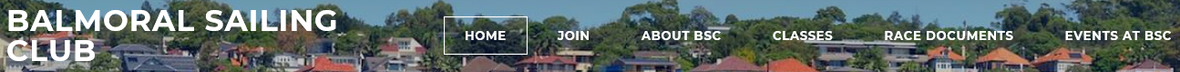Sneak peak...

A separate membership portal with individual logins for all members and online payment of membership and racking fees are also underway. The future's so bright!

## Electronic Sign On

Next season we will introduce electronic sign on. This replaces paper sign on in the clubhouse. The electronic form can be completed at home before the race or on your mobile device. There will also be tablets available at the clubhouse. We have tested the electronic form with a group of pilot users and are now fine tuning the process. Stay tuned!

Marco Teering, Director BSC

 table div table+table+table+table+table+table+table+table+table+table+table+table+table+table+table+table+table+table+table+table+table+table+table+table+table+table+table div table{width:100%;padding:0}table div table+table+table+table+table+table+table+table+table+table+table+table+table+table+table+table+table+table+table+table+table+table+table+table+table+table+table div table img{width:96.23%;padding:0;float:none}table div table+table+table+table+table+table+table+table+table+table+table+table+table+table+table+table+table+table+table+table+table+table+table+table+table+table+table div table td{width:100%;padding:0 1.88% 18px}/* styles */## National E NSW State Titles Report

 /* styles */ BSC hosted the National E States on 24th March. The weather was an ideal NE freshening from light to moderate over the afternoon's racing.
 /* styles */ We were fortunate to have two visiting boats from Victoria who may have thought they could win the title. Happily for NSW competitors the trophy remains secure here for another year.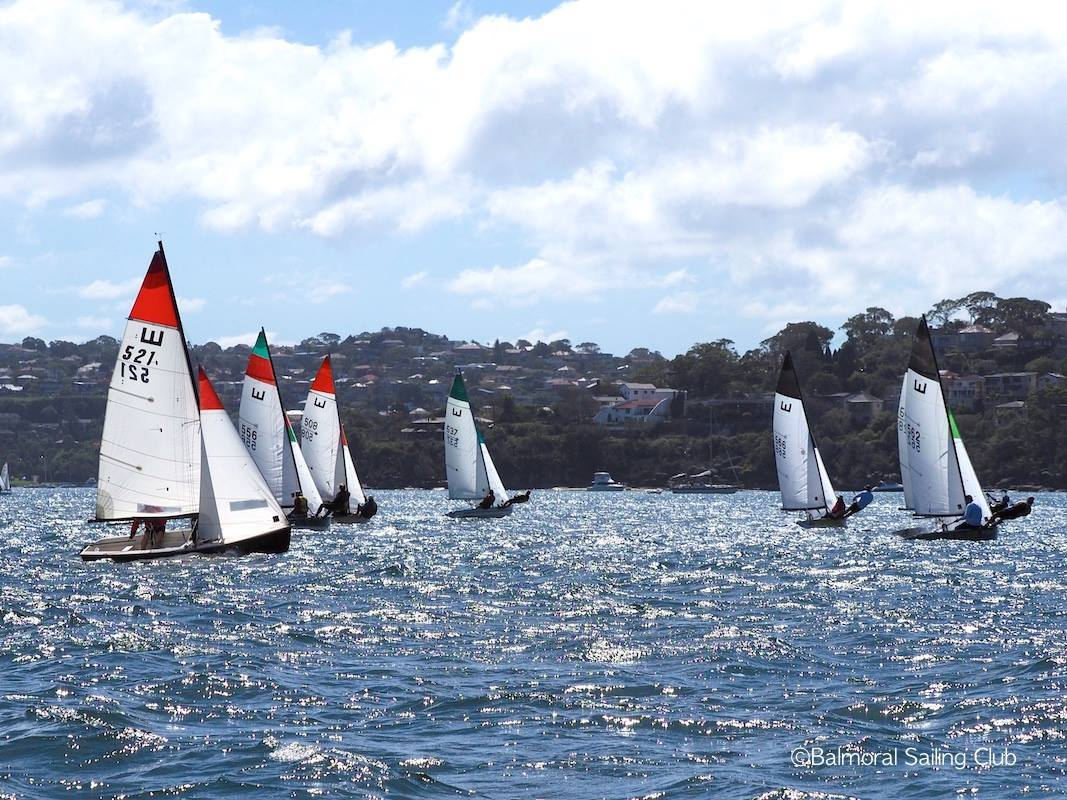(Photo: Rob Owe-Young)

For the first time National E sailed a three back to back race series which for the organisers (convener Peter Nixey and Race Officer Heather Hall) had potential problems. With a one hour limit, the regular courses had to be shortened dramatically to suit the time requirement.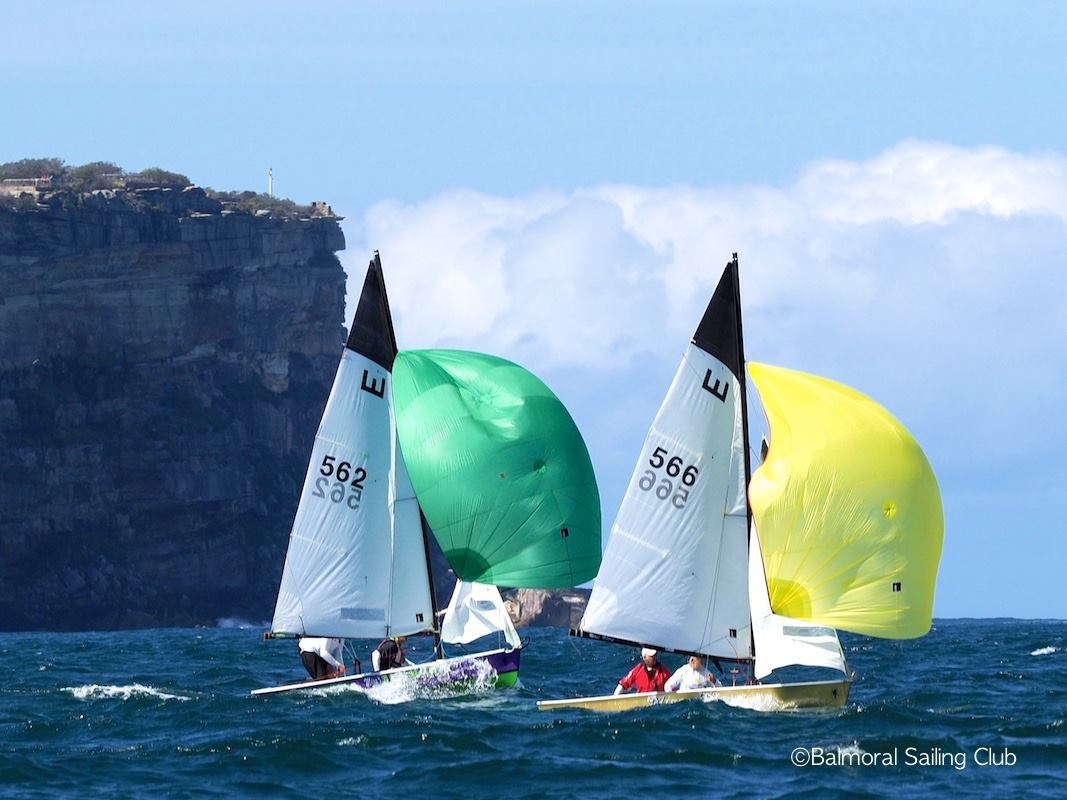(Photo: Rob Owe-Young)

Normal fixed positions for the start boat and mark rounding directions were needed as club racing took place simultaneously. These requirements meant a few last minute changes to the course sheets that were (we thought) dealt with adequately at the briefing.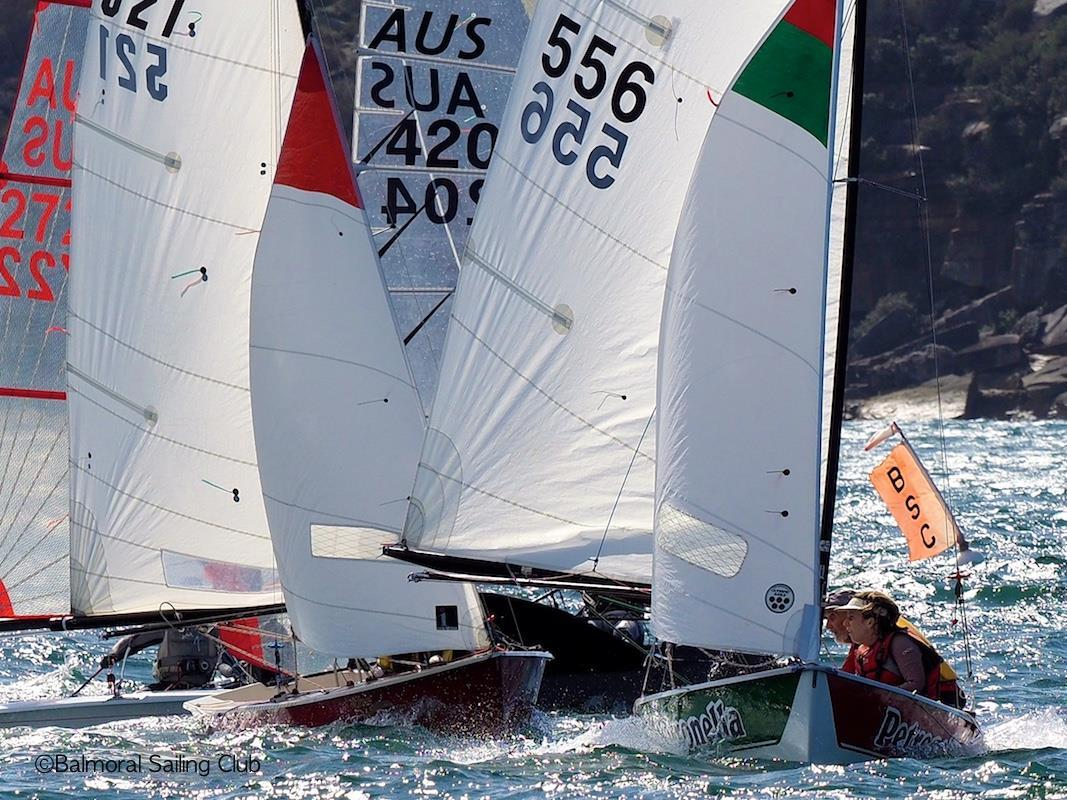(Photo: Rob Owe-Young)

Nevertheless old habits die hard and Escapee (leading boat and eventual winner) rounded No 2 in the wrong direction followed by another three boats. It was too bad that all four were disqualified but in fact Geoff and Sarah sailed Escapee so well over this event that they won regardless of the penalty!

## Final results of the National E 2018 NSW State Titles

Overall - Boat - Helm & Crew - Race 1/2/3 Points
1st - Escapee - Geoff Gill & Sarah Mckay - 8/1/1
2nd - Firewind - Dennis Patton & Peter Humphfey - 1/6/4
=3rd - Miss Adventure - Mark Foster & Joe Pecone - 8/2/3
=3rd - Miss Bling - Dan Osinga & Craig Finger - 8/3/2
5th - Fury - Peter Nixey & Murray Town - 2/5/7
6th - Costl-e - Ken Wilson & Tim Sussman - 3/7/6
7th - Petronella - Will Jones & Emily Jones - 8/4/5

 table div table+table+table+table+table+table+table+table+table+table+table+table+table+table+table+table+table+table+table+table+table+table+table+table+table+table+table+table+table+table+table+table+table+table+table+table div table{width:100%;padding:0}table div table+table+table+table+table+table+table+table+table+table+table+table+table+table+table+table+table+table+table+table+table+table+table+table+table+table+table+table+table+table+table+table+table+table+table+table div table img{width:96.23%;padding:0;float:none}table div table+table+table+table+table+table+table+table+table+table+table+table+table+table+table+table+table+table+table+table+table+table+table+table+table+table+table+table+table+table+table+table+table+table+table+table div table td{width:100%;padding:0 1.88% 18px}/* styles */## Get that Rash(ie) looked at

 /* styles */ Ever wondered why some members are so popular, attractive, intelligent and always on top of it? Research concluded that BSC apparel is the most important common factor in achieving success*.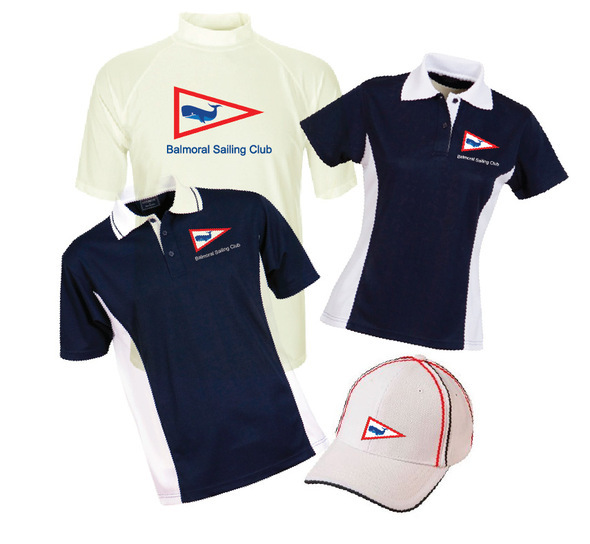A rashie you'll look good in.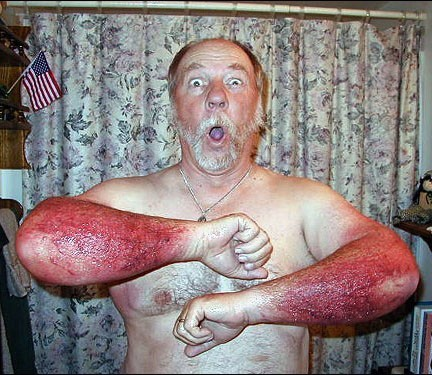A rash you should get looked at.

## Polo shirts

Members \$31.50
Non members \$41.50

Members \$60
Non members \$ 70

## Payments

Balmoral Sailing Club (co-op) Ltd
BSB: 633000
A/C no : 153560891

 /* styles */ Note: *Success refers to the marketing team coffers, not the wearer.
 table div table+table+table+table+table+table+table+table+table+table+table+table+table+table+table+table+table+table+table+table+table+table+table+table+table+table+table+table+table+table+table+table+table+table+table+table+table+table+table+table+table+table+table div table{width:100%;padding:0}table div table+table+table+table+table+table+table+table+table+table+table+table+table+table+table+table+table+table+table+table+table+table+table+table+table+table+table+table+table+table+table+table+table+table+table+table+table+table+table+table+table+table+table div table img{width:96.23%;padding:0;float:none}table div table+table+table+table+table+table+table+table+table+table+table+table+table+table+table+table+table+table+table+table+table+table+table+table+table+table+table+table+table+table+table+table+table+table+table+table+table+table+table+table+table+table+table div table td{width:100%;padding:0 1.88% 18px}/* styles */## Presentation Night - Saturday 19th May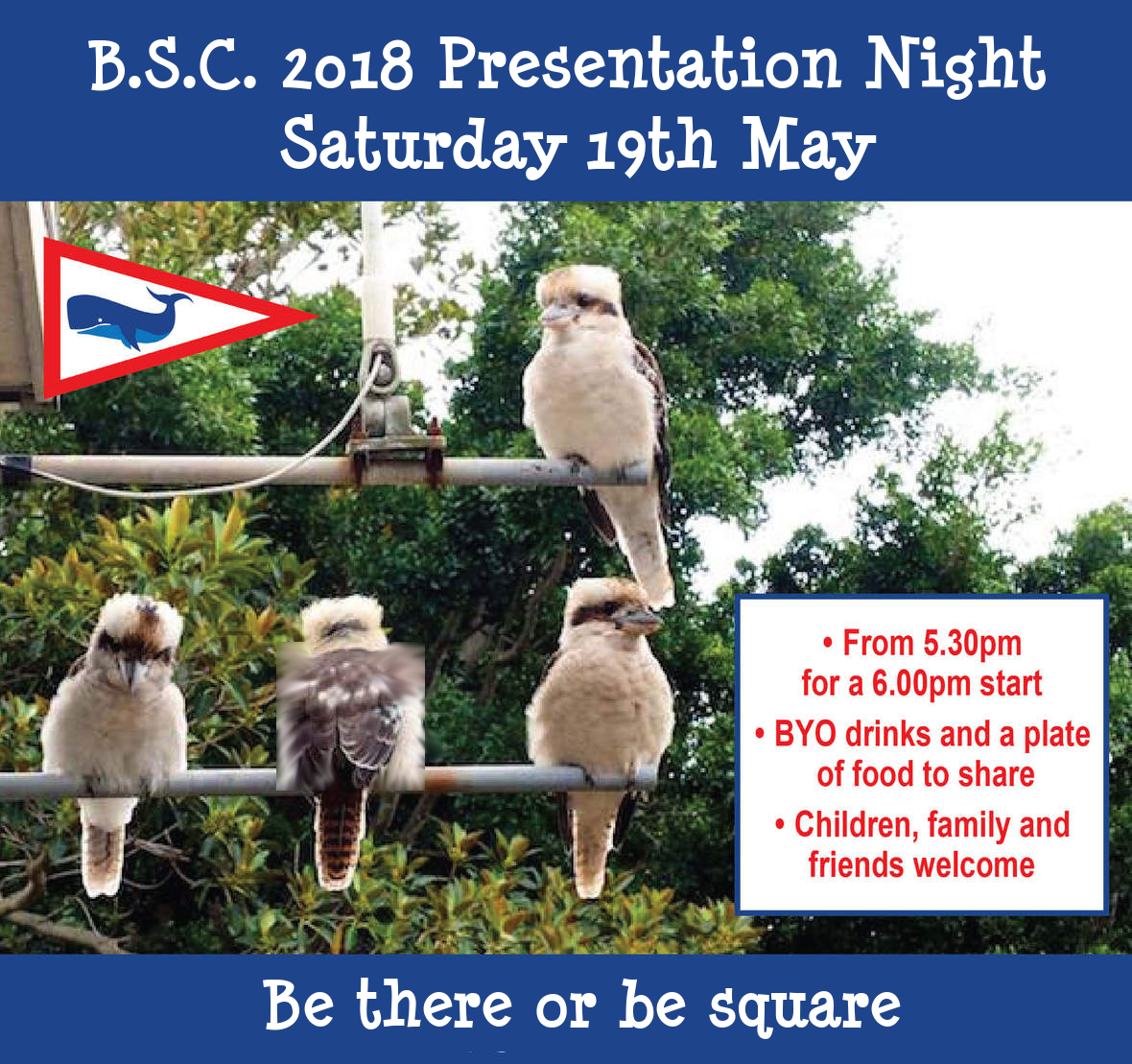3 Kookaburra will be there, the other...
 table div table+table+table+table+table+table+table+table+table+table+table+table+table+table+table+table+table+table+table+table+table+table+table+table+table+table+table+table+table+table+table+table+table+table+table+table+table+table+table+table+table+table+table+table+table+table+table div table{width:100%;padding:0}table div table+table+table+table+table+table+table+table+table+table+table+table+table+table+table+table+table+table+table+table+table+table+table+table+table+table+table+table+table+table+table+table+table+table+table+table+table+table+table+table+table+table+table+table+table+table+table div table img{width:96.23%;padding:0;float:none}table div table+table+table+table+table+table+table+table+table+table+table+table+table+table+table+table+table+table+table+table+table+table+table+table+table+table+table+table+table+table+table+table+table+table+table+table+table+table+table+table+table+table+table+table+table+table+table div table td{width:100%;padding:0 1.88% 18px}/* styles */## Premiere's Grant

 /* styles */ Last year we applied for a community grant to help with the cost of replacing the roof over bays C and D. This application was unsuccessful.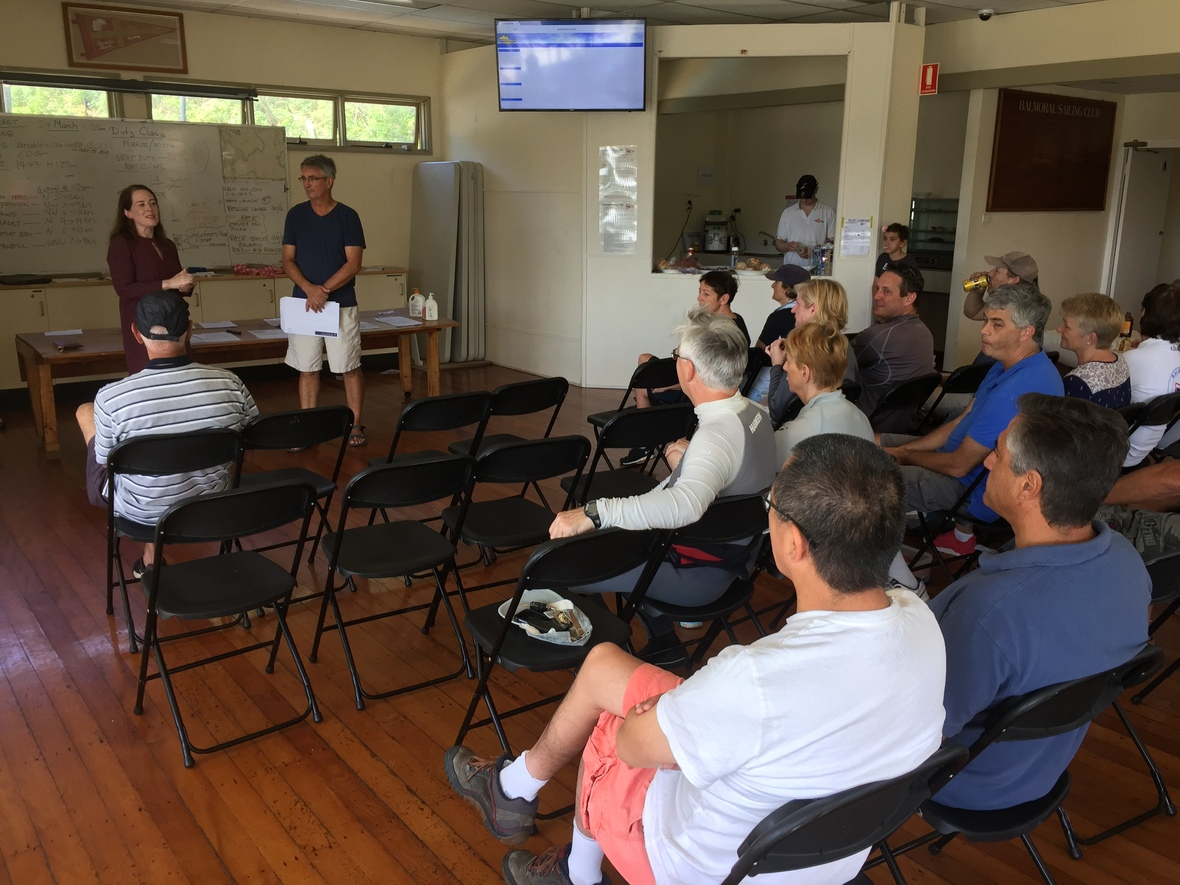Felicity Wilson MP, state member of parliament for the North Shore, came to see us again on March 24th. Following a request by Felicity, the Premier Gladys Bererjiklian provided the club a Premier's Grant of \$11,604 covering half the roof repair cost completed late last year.

 /* styles */ At our latest briefing Felicity Wilson presented Club Captain David Johnson with the grant and thanked the club for our work in the community.
 table div table+table+table+table+table+table+table+table+table+table+table+table+table+table+table+table+table+table+table+table+table+table+table+table+table+table+table+table+table+table+table+table+table+table+table+table+table+table+table+table+table+table+table+table+table+table+table+table+table+table+table+table div table{width:100%;padding:0}table div table+table+table+table+table+table+table+table+table+table+table+table+table+table+table+table+table+table+table+table+table+table+table+table+table+table+table+table+table+table+table+table+table+table+table+table+table+table+table+table+table+table+table+table+table+table+table+table+table+table+table+table div table img{width:96.23%;padding:0;float:none}table div table+table+table+table+table+table+table+table+table+table+table+table+table+table+table+table+table+table+table+table+table+table+table+table+table+table+table+table+table+table+table+table+table+table+table+table+table+table+table+table+table+table+table+table+table+table+table+table+table+table+table+table div table td{width:100%;padding:0 1.88% 18px}/* styles */## Think you have enough to worry about?

We battle in varying conditions, tweak equipment, employ a multitude of techniques and tactics, all in the knowledge that a simple rule infringement may prove all efforts to be futile.

Sailing is a challenging sport - it could be worse...

Ancraophobia - likely deal breaker.
Aquaphobia - no roll tack advice from Tasared Twice.
Asymmetriphobia - 16ft of terror.
Catoptrophobia - the perilous red sails.
Dextrophobia - for helms on starboard tacks.
Doxophobia - no crew ever suffered from this.
Enosiophobia - no helm need worry.
Frigophobia - the WSS ain't for you.
Hodophobia - the Traveler Trophy ain't for you either.
Leukophobia - thank goodness for Mylar.
Levophobia - for crews on starboard tacks.
Mottephobia - the silent foiling menace.
Triskaidekaphobia - well it is very close to Mark 2.

If you sail and suffer any of these then I doff my hat to you - with apologies to those with Cocklaphobia.

 table div table+table+table+table+table+table+table+table+table+table+table+table+table+table+table+table+table+table+table+table+table+table+table+table+table+table+table+table+table+table+table+table+table+table+table+table+table+table+table+table+table+table+table+table+table+table+table+table+table+table+table+table+table+table+table div table{width:100%;padding:0}table div table+table+table+table+table+table+table+table+table+table+table+table+table+table+table+table+table+table+table+table+table+table+table+table+table+table+table+table+table+table+table+table+table+table+table+table+table+table+table+table+table+table+table+table+table+table+table+table+table+table+table+table+table+table+table div table img{width:96.23%;padding:0;float:none}table div table+table+table+table+table+table+table+table+table+table+table+table+table+table+table+table+table+table+table+table+table+table+table+table+table+table+table+table+table+table+table+table+table+table+table+table+table+table+table+table+table+table+table+table+table+table+table+table+table+table+table+table+table+table+table div table td{width:100%;padding:0 1.88% 18px}/* styles */## Important Dates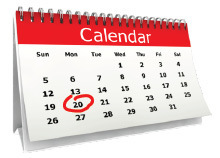19 May : BSC Presentation Night
30 June : Rescue Training Day
18-25 July : Tasar Nationals
5 Aug : AGM & Working Bee
26-30 Dec : Mirror Nationals
31 Dec - 6 Jan: Mirror Worlds

 /* styles */ And don't forget post-race sausage sizzles are on every week. Sailing is great but there is one snag (or maybe two if you're hungry).